# Stability

As you have probably noticed, my knowledge base articles are free of advertising. Instead of distracting you with annoying ads, I kindly request your donation. If you find the contents of this page to be useful, please consider making a donation by clicking the Donate button below.Stability: Section Outline

The main source of concern of the DIY crowd appears to be stability. There are good reasons for this. High frequency instability can cause the amplifier to emit large signals at frequencies well above the audible range for humans. These signals will dissipate significant power in the speaker tweeters, causing them to fail. Hence, many amps have been given a bad reputation for being "tweeter eaters". This is further exacerbated by the fact that many DIY enthusiasts do not own the tools, such as oscilloscopes and signal generators, to spot the signs of instability before the speakers are connected.

I have identified five main causes of high frequency instability:

• Low phase or gain margin
• Excessive parasitic inductance in the feedback network
• Lack of supply decoupling
• Operation without Zobel or Thiele networks

To determine the impact of each of these culprits, I rely on circuit simulations validated by laboratory measurements. Simulations have the beautiful feature that circuit performance under ideal conditions can be compared with circuit performance under real conditions by including the relevant parasitics in the simulation.

Model Validation

For the simulations, I chose to use TINA-TI, a free Spice circuit simulator available from Texas Instruments. I chose TINA because it is directly compatible with the Spice models found on TI's website. Note that TINA uses modern day SI notation for the prefixes. I.e., m = 10-3 = milli and M = 106 = mega, unlike Spice which is not case sensitive and uses "meg" for mega.

The first step with any simulation project is to validate the models used. In other words, simulation results need to be compared with reality. As the objective of this project is to determine the stability of an LM3886-based amplifier, the primary point of interest is the open-loop gain/phase curve. This is simulated below.The challenge with open-loop measurements (and simulations) is that the feedback loop needs to be closed at DC so the circuit can settle at the correct DC operating point, but needs to be opened for the AC part of the measurement or simulation. In simulation, this is easy. An LC lowpass filter (L1, C1) with an incredibly low cutoff frequency is inserted in the feedback loop. The cutoff frequency of the filter is lowered until it no longer impacts the gain/phase response within the frequency band of interest. In this case, an LC filter using L1 = 10 MH and C1 = 10 MF is used. This results in a cutoff frequency of 16 nHz. Gotta love simulation... The resulting gain/phase plot is compared with the data sheet figure:Simulation shows a phase margin (PM) of 78 ° for a gain of 20 dB (10x). The data sheet indicates a PM of about 65 °. Simulation also shows the unity-gain bandwidth (UGBW) to be about 10 MHz, whereas, the data sheet indicates an UGBW of about 4 MHz. The simulated DC gain (AVOL(DC)) matches the data sheet figure of 115 dB (TYP).

The skeptics are muttering in the hallway. "Yeah, yeah... All this simulation is great. What about reality?!" To address this, I measured the phase margin of an LM3886 amplifier. The amp was built using a very compact point-to-point layout and loaded by an 8 Ω resistor. The phase margin was measured using an HP 3577A network analyzer injecting a test signal into the feedback loop using a Tektronix CT-6 current transformer. The result is shown below. The marker is placed at the unity gain frequency.The CT-6 is optimized for use at RF, hence has significant roll-off near DC. This is responsible for the flattening of the gain and phase curves towards 200 kHz in the plot above. The phase margin measures 67.8 °. This is in very good agreement with the data sheet specs. The unity gain frequency measures 1.4 MHz, a bit higher still than the simulations and data sheet would indicate.

Conclusion: Overall, the model is slightly optimistic. The PM and UGBW are slightly higher than the data sheet indicates. The DC performance, however, is spot on. I trust the model to provide good information regarding trends and stability mechanisms and I would expect the simulated PM to be about 15~20 ° higher than the measured value. It is worth noting that the UGBW measures even higher on actual circuits than the simulated value.

The Influence of Circuit Layout on Stability

As noted in the LM3886 data sheet (p. 20), the inductance in the output lead of the amplifier IC greatly impacts stability. As a rule of thumb, figure that 1 mm of wire or PCB trace has a self-inductance of approx. 1 nH. Hence, the placement of the feedback resistor will have a big impact on amplifier performance.

The example below shows two common layouts of the LM3886 with resistive feedback. Only the feedback resistors have been included here. The dimensions marked in green are in mil (1 mil = 0.001" = 25.4 µm).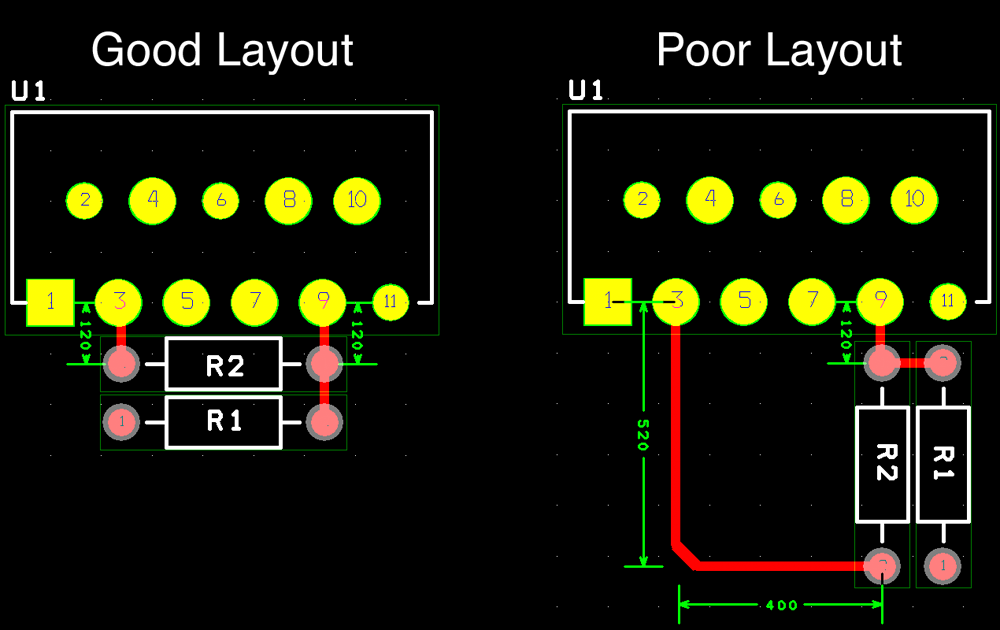As shown, the good layout results in approx. 240 mil (6.1 mm) of trace routing in the feedback network. Allowing for a little parasitic inductance in resistor R2, the parasitic inductance in the feedback path of the good layout is approx. 10 nH.

The poor layout exhibits 1.04" (26.4 mm) of trace routing, resulting in a parasitic inductance of roughly 30 nH.

Simulations of the phase margin (PM) and gain margin (GM) were performed for a parasitic inductance, Lout, of 0 nH (ideal case), 10 nH (good layout), and 30 nH (poor layout). The results are plotted below.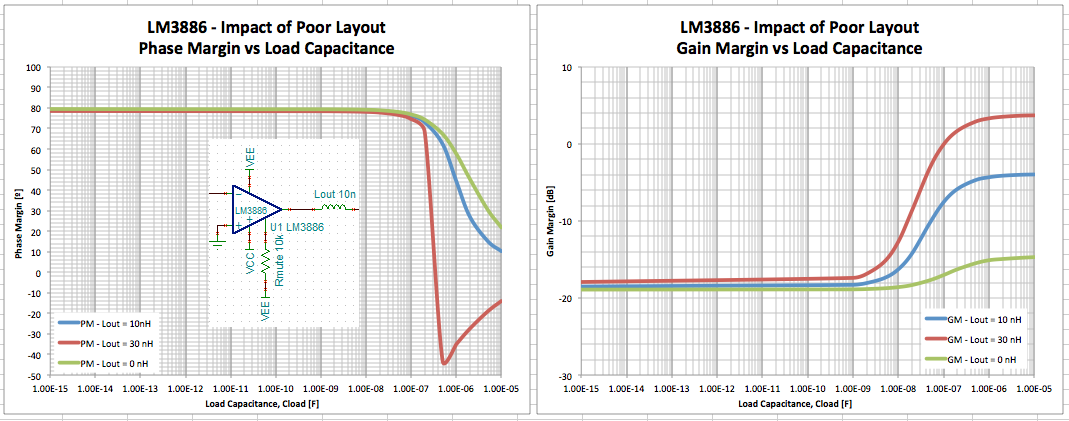Note that the textbook definition of stability is that PM > 0 ° and GM < 0 dB. In an actual circuit, I prefer the PM to be greater than 60 ° and the GM below -10 dB.

The impact of the layout is pretty stark. Note how the poor layout (which really does not look that bad in the layout editor) causes the PM to fall off a cliff once the load capacitance exceeds 200 nF. The degradation in circuit stability is caused by the resonance of Lout and Cload. It's pretty easy to spot the trouble brewing in the loop response.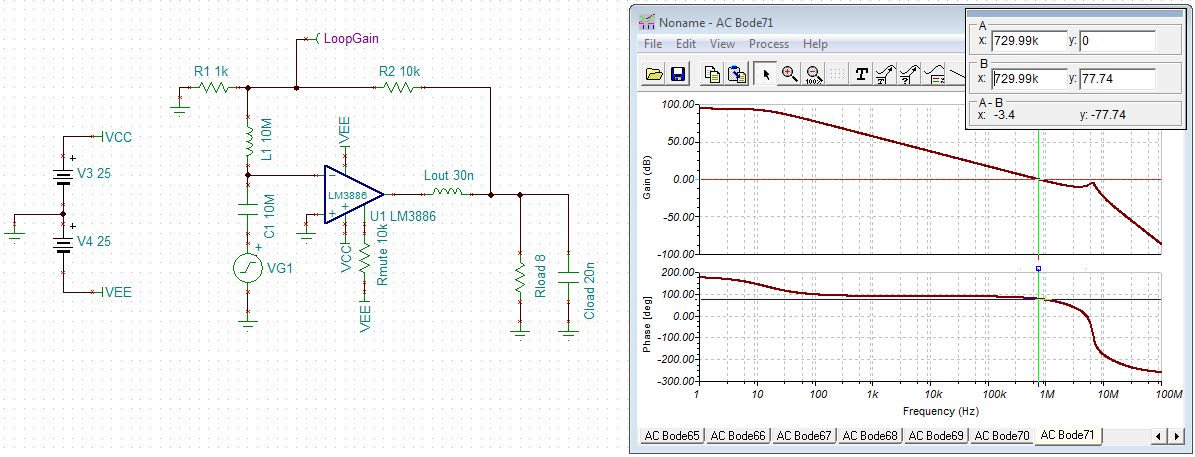Note the resonance at 6.5 MHz. Once that peak crosses 0 dB, the circuit will oscillate. This is a tweeter eater.

The Zobel Network

To combat the phase change caused by the Lout, Cload resonant circuit, a compensation network is added. Two types of networks are commonly used: an RC series combination from the amp output to ground and an LR parallel combination in series with the output. The resulting schematic is shown below. The values used are the ones listed in the LM3886 data sheet.

There is much confusion about the naming conventions of these output filters. Technically, the RC series network is the Zobel network and LR parallel combination a Thiele network. Occasionally, the Zobel network is referred to as a Boucherot cell as well. In the schematic below, it would have been more consistent with the naming convention to use Lt and Rt rather than Lz and Rz2. As previously stated, there's much confusion... I will do my best to limit the confusion from here on.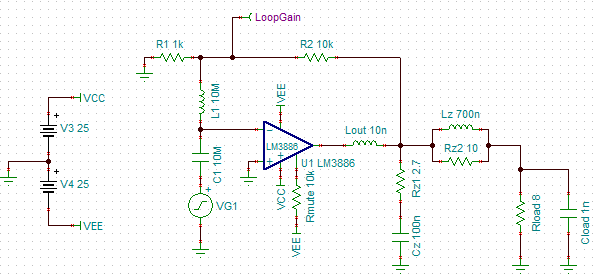The Zobel network performs two main functions:

1. If selected properly, it aids the stability of the LM3886 feedback loop by adding a pole-zero pair in the feedback loop. The pole-zero pair is the result of the frequency-dependent voltage divider comprised of the open-loop output impedance of the LM3886, Rout, together with Cz and Rz1.
2. It provides a low load impedance for the LM3886 output stage at frequencies where the Thiele network has effectively decoupled the external load impedance.

The LM3886 has a quasi-complimentary output stage. When the amplifier output is positive, the output is driven by an emitter follower. When output is negative, a complimentary feedback pair (CFP) output stage drives the output.

Emitter followers are notorious for oscillating under light load conditions. This is caused by the rolloff of transistor current gain (β) at HF. In addition the maximum operating speed of the transistor, ft, decreases at light load. These two properties cause the output impedance of the emitter follower to be inductive at HF, in particular at light load. The output inductance forms a resonant circuit with the parasitic and load capacitance, resulting in oscillations. This is combated effectively by adding a load, Rz1, to the amplifier. To avoid dissipating significant power at DC, a capacitor, Cz, is in series with the resistor.

CFP output stages contain a local feedback loop with relatively high gain. This is a common culprit for oscillations as well, which is why the LM3886, under marginal stability conditions, will often oscillate on the negative swing but not the positive. On the CFP output, the Zobel network, essentially, works as a snubber, dampening the local oscillations.

The main function of the Thiele network is to isolate the external load capacitance, Cload, from the amplifier output at HF.

Rerunning the PM, GM vs Cload simulation with the Zobel and Thiele networks added, the following results are obtained.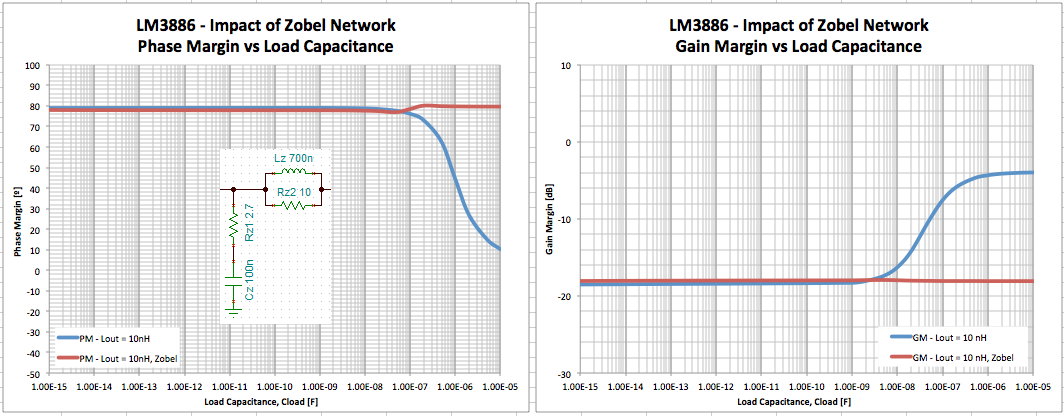The importance of the Zobel and Thiele networks is pretty obvious here... The improvement is mainly due to the Thiele network. Let's explore that a bit from an intuitive standpoint. There are three main frequency regions of interest:

1. Low frequency: Audio frequencies. At these frequencies, the impedance of Cz is significantly greater than that of Rz1. At these frequencies, the Zobel network is not presenting much of a load on the amplifier. Consider it an open circuit. For the Thiele network, at LF, the impedance of Lz is significantly smaller than that of Rz2, so the parallel combination of the two is dominated by Lz. Hence, Lz || Rz2 present practically a short circuit at LF.
2. High frequency: At HF, the impedance of Cz is significantly smaller than Rz1, hence, the Zobel network is mostly resistive. It will present a load of Rz1 = 2.7 Ω on the amplifier. The impedance of the Thiele network is dominated by Rz2 as the inductor's impedance is significantly higher than Rz2 at HF. The total load on the LM3886 will be Rz1 in parallel with Rz2 + Zload. If Zload is mainly capacitive, it will act like a short circuit at HF. Thus, the total load on the LM3886 approaches Rz1 || Rz2 as the frequency approaches infinity.
3. The transition region. This is the region where LF transitions to HF. The component values for the Thiele and Zobel networks should be selected to place the transition region well outside the audio band.

So what is considered "LF" and "HF"? Numbers, please! Notice the use of the word 'significantly' in sections 1. and 2. above? What is 'significant'? In most engineering math, where 1-2 digit precision is the norm and three digits considered a luxury, "significantly greater" means "a factor of ten greater". I would go as far as saying that 5~10x is probably close enough.

From 1. and 2. above, the transition region is the frequency range where neither Rz1, Cz or Rz2, Lz dominate over one another. Recall, the reactance of a capacitor and an inductor are: XC = 1/(2π·f·C) and XL = 2π·f·L, respectively. The frequency where the reactance of Cz is considered significantly greater than Rz1 can be found by solving this equation: 10·Rz1 = 1/(2π·f·Cz). Solving for f yields: f = 1/(2π·C·10·Rz1). Similarly for the Thiele network: Rz2 = 10·2π·f·Lz → f = Rz2/(2π·10·Lz). Plugging in the values from the schematic above yields: 1/(2π·C·10·Rz1) = 58.9 kHz and Rz2/(2π·10·Lz) = 227 kHz respectively. Hence, the lower bound on the transition region would be 58.9 kHz.

Substituting 0.1 for 10 in the equations above yields the upper bound on the transition band: 1/(2π·C·0.1·Rz1) = 5.89 MHz; Rz2/(2π·0.1·Lz) = 22.7 MHz.

From these calculations, it is determined that frequencies from DC to 59 kHz should be considered "LF", frequencies above 23 MHz, "HF", and the range in between, the transition band.

Some designers will use two Zobel networks. One located close to the IC and one mounted on the speaker output terminals of the amplifier. However, simulation shows minimal impact of the second Zobel network.The inductance L2 and resistance R4 model the effect of the wire between the amplifier board and the speaker terminals. I figured 15 cm (150 nH) of wire would be representative of a nice, compact layout.

Note: The power dissipated in Rz1 depends on the capacitance of Cz: P(Rz1) = f·Cz·V². The voltage V is the RMS output voltage at output frequency f. A few examples are tabulated below.

Rail Voltage Cz Rz1 P(Rz1)
±22 V 100 nF 2.7 Ω 416 mW
±28 V 100 nF 2.7 Ω 697 mW
±35 V 100 nF 2.7 Ω 1.12 W
±28 V 56 nF 4.7 Ω 390 mW
±28 V 33 nF 8.2 Ω 230 mW

Above calculations assume a symmetric drop-out voltage of 1.6 V at clipping. Note that all RC combinations listed form a zero at roughly the same frequency, hence, are equally effective as a Zobel network as far as stability is concerned, however, they may not all be equally effective as an HF load for the LM3886. For continuous sine wave operation, the resistor Rz1 should be rated for operation at 3~4 times higher power than listed above. For music signals with crest factors of 3 dB or higher, one can probably get away with specifying a power dissipation rating for Rz1 of 1~2 times the value calculated above. For the higher rail voltages it is good practice to choose a Cz1 with a lower capacitance such that a resistor with a lower power rating can be used. Note, however, that power resistors of higher resistances tend to exhibit higher parasitic inductance which will affect the frequency response of the Zobel network. I suggest limiting Rz1 to 10 Ω to avoid excessive inductance. Alternatively, a thick film resistor type can be chosen.

Those Extra "Stability Components"

Much is read into the various figures in the data sheet. For example, Rf2, Cf, and Cc in the AC Electrical Test Circuit are often mentioned by DIYers as "required for stability". Note that these test circuits are optimized for use on the tester used for final test. These testers and load boards often exhibit significant parasitics, hence, these circuits should not necessarily be considered "recommended" or "required" configurations.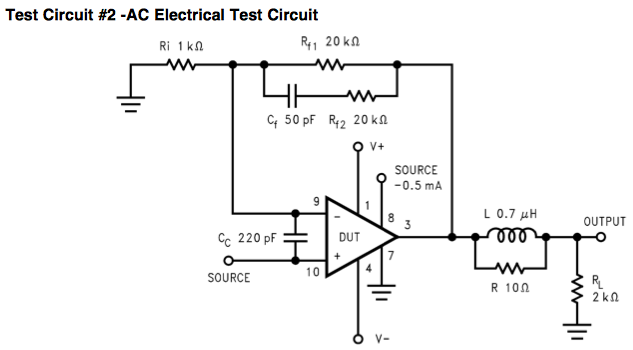The circuit works as follows: Rf1 and Ri set the LF gain to +21 V/V. At HF (when the reactance of Cf is small compared to Rf2), the gain is set by (Rf1 || Rf2) and Ri to +11 V/V. This creates a zero in the loop gain response around 150 kHz followed by a pole at about 300 kHz. This explains the slight bump around 200 kHz on the gain curve in the simulation below.Note that the phase margin is actually considerably worse than without those "stability components". Here is the circuit without the "stability components":And phase/gain margins versus load capacitance: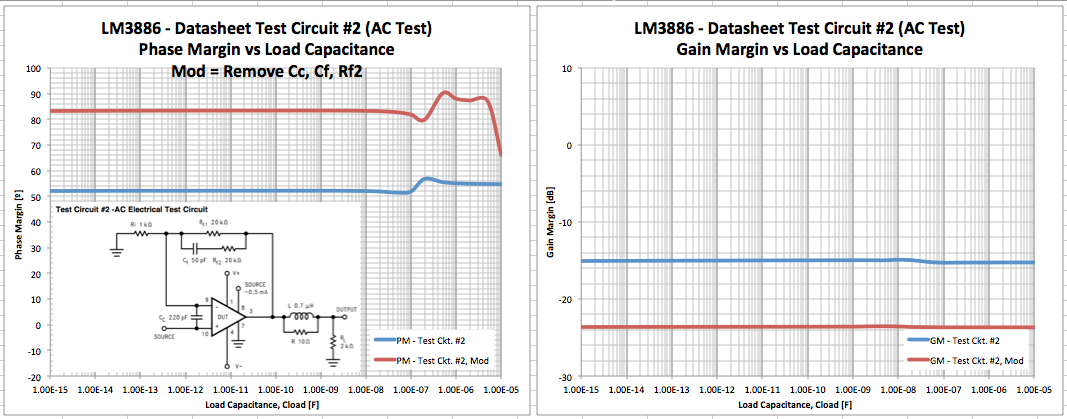The degradation in phase/gain margin is primarily caused by Cc. According to the data sheet (pp. 8, 22), the purpose of Cc is to eliminate quasi oscillation caused by the pickup of EMI from the engagement of the SPiKe over-current protection. Cc is needed if the output impedance of the source driving the LM3886 is high and long signal traces are used. It follows that the quasi oscillation issue could just as well be addressed by lowering the source impedance or placing a resistor from the non-inverting input pin to ground on the LM3886. The EMI filter can then be placed on the non-inverting input of the LM3886, where it does not affect the phase margin.

It should be noted that it is possible that Rf2 and Cf are employed to address effects not included in the model. Stay tuned for lab measurements.

Section 2: Supply Decoupling